# Trix (technical analysis)

Trix (or TRIX) is a technical analysis oscillator developed in the 1980s by Jack Hutson, editor of Technical Analysis of Stocks and Commodities magazine. It shows the slope (i.e. derivative) of a triple-smoothed exponential moving average. The name Trix is from "triple exponential."

Trix is calculated with a given N-day period as follows:

• Smooth prices (often closing prices) using an N-day exponential moving average (EMA).
• Smooth that series using another N-day EMA.
• Smooth a third time, using a further N-day EMA.
• Calculate the percentage difference between today's and yesterday's value in that final smoothed series.

Like any moving average, the triple EMA is just a smoothing of price data and, therefore, is trend-following. A rising or falling line is an uptrend or downtrend and Trix shows the slope of that line, so it's positive for a steady uptrend, negative for a downtrend, and a crossing through zero is a trend-change, i.e. a peak or trough in the underlying average.

The triple-smoothed EMA is very different from a plain EMA. In a plain EMA the latest few days dominate and the EMA follows recent prices quite closely; however, applying it three times results in weightings spread much more broadly, and the weights for the latest few days are in fact smaller than those of days further past. The following graph shows the weightings for an N=10 triple EMA (most recent days at the left):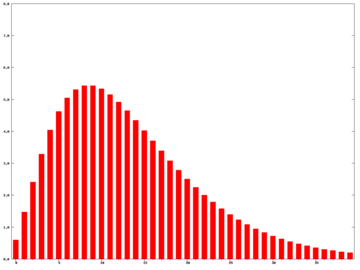Triple exponential moving average weightings, N=10 (percentage versus days ago)

Note that the distribution's mode will lie with pN-2's weight, i.e. in the graph above p8 carries the highest weighting. An N of 1 is invalid.

The easiest way to calculate the triple EMA based on successive values is just to apply the EMA three times, creating single-, then double-, then triple-smoothed series. The triple EMA can also be expressed directly in terms of the prices as below, with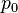$p_0$ today's close,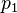$p_1$ yesterday's, etc., and with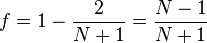$f = 1 - {2\over N+1} = {N-1\over N+1}$ (as for a plain EMA):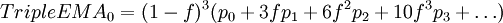$TripleEMA_0 = (1-f)^3 (p_0 + 3fp_1 + 6f^2p_2 + 10f^3p_3 + \dots)$

The coefficients are the triangle numbers, n(n+1)/2. In theory, the sum is infinite, using all past data, but as f is less than 1 the powers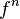$f^n$ become smaller as the series progresses, and they decrease faster than the coefficients increase, so beyond a certain point the terms are negligible.